# transectc documentation

transectc produces a contour plot oceanographic data from CTD profiles collected at different locations and/or times.

See also: transect for help converting data to cell arrays.

## Syntax

```transectc(x,d,v)
transectc(...,levels)
transectc(...,LineSpec)
transectc(...,'Name',Value)
transectc(...,'extrap')
transectc(...,'interp','InterpMethod)
transectc(...,'di',di)
transectc(...,'xi',xi)
[C,h] = transectc(...)```

## Description

transectc(x,d,v) creates a contour plot diagram of the variable v at the horizontal locations (or times) x and depths d. x must be a numeric array of values indicating the locations or times of each CTD profile. Inputs d and v must each be cell arrays containing depths and measurements at each CTD location.

transectc(...,levels) specifies the values of v to draw as contour lines. If levels is a scalar value, then transectc draws that number of contour lines. If levels is an array, a contour line is plotted for each value in levels. To draw the contours at one value (k), specify levels as a two-element row vector [k k].

transectc(...,LineSpec) specifies the style and color of the contour lines.

transectc(...,'Name',Value) specifies additional options for the contour plot using one or more name-value pair arguments. Specify the options after all other input arguments. For a list of properties, see Contour Properties.

transectc(...,'extrap') turns on the option to extrapolate beyond the extents of data. By default, transectc does not extrapolate, but the option is available to fill in gaps, such as between lowest datapoint and the seabed.

transectc(...,'interp','InterpMethod) specifies an interpolation method used for the contouring. Default is 'linear'.

transectc(...,'di',di) specifies interpolation depths di. By default, transectc creates a grid by interpolating to 1000 equally-spaced depths between the shallowest and deepest measurement.

transectc(...,'xi',xi) specifies interpolation locations (or times) xi. By default, transectc interpolates to 2000 equally-spaced points xi between the minimum and maximum values of x.

[C,h] = transectc(...) returns the contour matrix C and the handle h of the plotted objects.

## Methods

This function grids unevenly spaced data by the method described in the documentation for the transect function, then plots the gridded data with Matlab's built-in contour function.

## Examples

The examples below use some Argo data that you can load like this:

```load example_ctd

whos % displays the names and sizes of each variable
```
```  Name      Size            Bytes  Class     Attributes

P         1x95            25152  cell
S         1x95            25152  cell
T         1x95            25152  cell
bed       1x95              760  double
x         1x95              760  double

```

The Argo data contain 95 profiles taken as the float drifted along. Each profile was taken at a location in x, the corresponding bed depths are given by bed and the temperatures T and salinities S are in cell arrays at the corresponding pressures P. See transect for help converting data into cell arrays.

## Example 1: Simplest form

Here's a simple transect of temperature data T taken at locations x and depths P:

```transectc(x,P,T)

colorbar
xlabel 'distance along transect (km)'
ylabel 'pressure (dbar)'
```## Example 2: Specify contour levels with different line styles

Like above, but now only plot negative values, with lines every 0.1 degrees:

```vals = -2:0.1:0;

figure
transectc(x,P,T,vals)

colorbar
xlabel 'distance along transect (km)'
ylabel 'pressure (dbar)'
```Now only plot negative values and make them black dashed lines:

```figure

transectc(x,P,T,vals,'k--')

xlabel 'distance along transect (km)'
ylabel 'pressure (dbar)'
```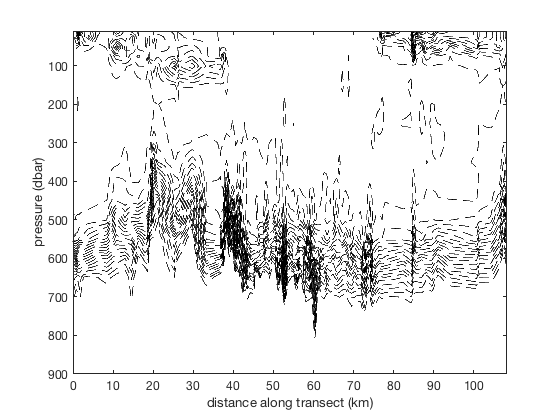and positive values have dotted lines:

```hold on
transectc(x,P,T,0:0.1:2,'k:')
```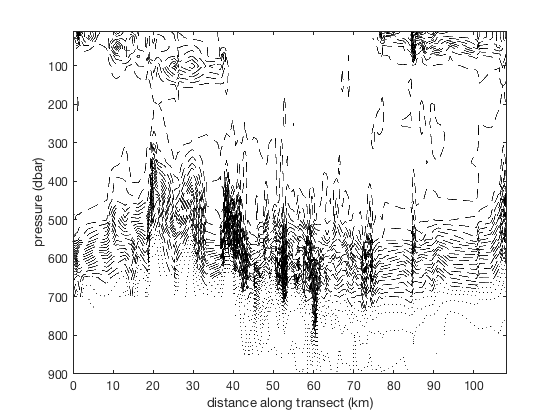and the zero contour is thicker:

```transectc(x,P,T,[0 0],'color',rgb('deep green'),'linewidth',3)
```## Example 3: Use in conjunction with transect

A contour plot can be difficult to interpret on its own. When used in conjunction with the transect function, it can illustrate important changes in properties. See documentation for transect for an explanation of the options.

```figure
transect(x,P,T,...
'bed',bed,...        % add bedrock data
'bed',bed,'bedcolor',rgb('brown'),... % colour the bedrock brown
'marker','none',...      % turn off markers
'extrap')

caxis([-1.9 0.9])          % sets color axis limits

xlabel 'distance along transect (km)'
ylabel 'pressure (dbar)'
cmocean thermal            % sets the colormap
cb = colorbar;
ylabel(cb,'temperature \circC')
```Now add contours, making sure to suppress the output from printing to screen:

```hold on
[C,h] = transectc(x,P,T,-2:0.1:0);
```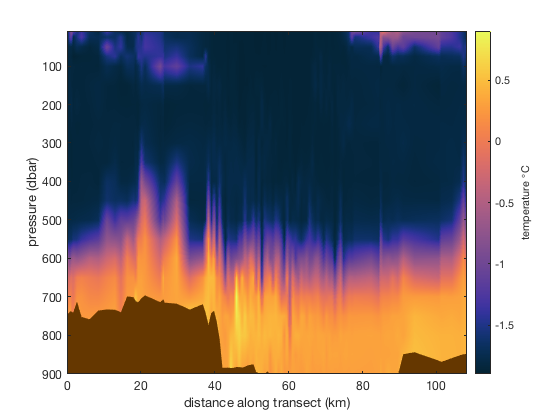Note that they're not visible, as they've taken the same colour map as the transect plot below. To make them visible, we'll need to give them a constant colour. Add positive contours as dotted lines and dashed lines as negative contours, with the zero temperature contour being a thick white line:

```delete(h) % deletes contours from above

transectc(x,P,T,-2:0.25:0,'k--')
```and positive values have dotted lines:

```transectc(x,P,T,0:0.25:2,'k:')
```and the zero contour is thicker:

```transectc(x,P,T,[0 0],'w','linewidth',3)
```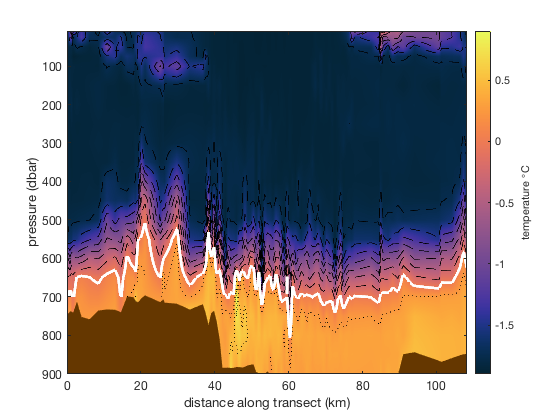Do the same again, but this time add text labels to each contour.

```figure
transect(x,P,T,...
'bed',bed,...        % add bedrock data
'bed',bed,'bedcolor',rgb('brown'),... % colour the bedrock brown
'marker','none',...      % turn off markers
'extrap')

caxis([-1.9 0.9])          % sets color axis limits

xlabel 'distance along transect (km)'
ylabel 'pressure (dbar)'
cb = colorbar;
ylabel(cb,'temperature \circC')
cmocean thermal % sets colormap
```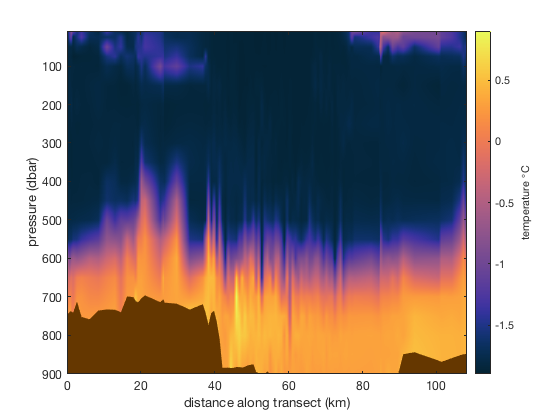Now add text labels by setting the 'ShowText' option, with appropriate spacing between labels:

```hold on
transectc(x,P,T,-2:.25:2,'k-',...
'ShowText','on',...
'LabelSpacing',1000);
```## Author Info

This function is part of the Climate Data Toolbox for Matlab. The function and supporting documentation were written by David E. Gwyther of University of Tasmania, and Chad A. Greene of the University of Texas at Austin.